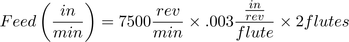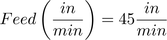# Speed/Feed Examples

## Milling Speed/Feed Example

Problem:

Calculate the cutting speed and feed for a milling operation given the following values:

 Parameter Value Tool Diameter .500 in NumFlutes 4 SFM 600 ft/min IPR .005 in

Solution:

Step 1: Calculate RPM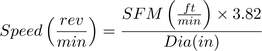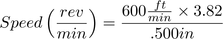Step 2: Calculate IPM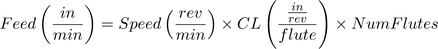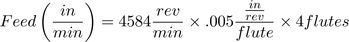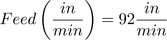Note: Round off milling speeds and feeds to the nearest integer.

## Drill Speed/Feed Example

Problem:

Calculate the cutting speed and feed for a drill operation given the following values:

 Parameter Value Tool Diameter .201 in SFM 250 ft/min IPR .002 in

Solution:

Step 1: Calculate RPM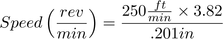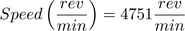Step 2: Calculate IPM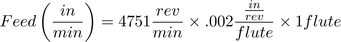Note: Round off drilling feeds to the first decimal point.

## Tap Speed/Feed Example

Problem:

Calculate the cutting speed and feed for a ¼-24 tap operation given the following values:

 Parameter Value Tool Diameter .25 in SFM 100 ft/min TPI 24

Solution:

Step 1: Calculate RPM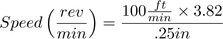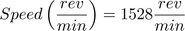Step 2: Calculate IPM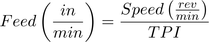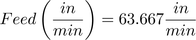Note: Round off tapping feeds to three decimal points or the maximum number the machine allows.

## Maximum Spindle Speed Example

In cases where the calculated spindle speed exceeds the machine capabilities, program the maximum spindle speed of the machine and use this value in the feed calculation.

Problem:

Calculate the cutting speed and feed for a milling operation given the following values:

 Parameter Value Tool Diameter .125 in NumFlutes 2 SFM 300 ft/min IPR .003 in/rev Maximum RPM 7,500 rev/min

Solution:

Step 1: Calculate RPM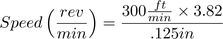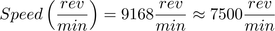Step 2: Calculate IPM